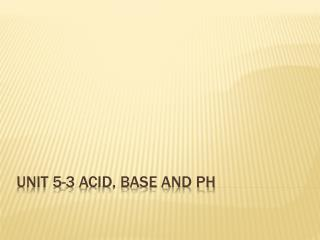DownloadDownload PresentationUnit 5-3 Acid, Base and PH

# Unit 5-3 Acid, Base and PH

Télécharger la présentation## Unit 5-3 Acid, Base and PH

- - - - - - - - - - - - - - - - - - - - - - - - - - - E N D - - - - - - - - - - - - - - - - - - - - - - - - - - -
##### Presentation Transcript

1. Unit 5-3 Acid, Base and PH

2. Arrhenius Model • Acids produce hydrogen ions in aqueous solutions • Bases produce hydroxide ions in aqueous solutions • Bronsted-Lowry Model • Acids are proton donors • Bases are proton acceptors Acid/Base Definitions

3. If it can be either… ...it is amphiprotic. HCO3− HSO4− H2O

4. What Happens When an Acid Dissolves in Water? • Water acts as a Brønsted–Lowry base and abstracts a proton (H+) from the acid. • As a result, the conjugate base of the acid and a hydronium ion are formed.

5. Conjugate Acids and Bases: • From the Latin word conjugare, meaning “to join together.” • Reactions between acids and bases always yield their conjugate bases and acids.

6. Acid and Base Strength • Strong acids are completely dissociated in water. • Their conjugate bases are quite negligible. • Weak acids only dissociate partially in water. • Their conjugate bases are weak bases, but stronger than the strong acids conjugate bases.

7. Acid and Base Strength • Substances with negligible acidity do not dissociate in water. • Their conjugate bases are exceedingly strong.

8. Strong Acids • You will recall that the seven strong acids are HCl, HBr, HI, HNO3, H2SO4, HClO3, and HClO4. • These are, by definition, strong electrolytes and exist totally as ions in aqueous solution. • For the monoprotic strong acids, [H3O+] = [acid].

9. Strong Bases • Strong bases are the alkali metal and heavier alkaline earth metal hydroxides (Ca2+, Sr2+, and Ba2+). • Strong bases are all soluble in water. • Again, these substances dissociate completely in aqueous solution. • MOH(s)  M+(aq) + OH-(aq)

10. Acid and Base Strength In any acid-base reaction, the equilibrium will favor the reaction that produces weaker acid and base. HCl(aq) + H2O(l) H3O+(aq) + Cl−(aq) • H2O is a much stronger base than Cl−, so the equilibrium lies so far to the right, Kc is very big. • For strong acid and base, when dissolving in water, the Kc is so big that the dissociation is considered irreversible.

11. Strong acids are assumed to dissociate completely in solution. Dissociation of Strong Acids

12. HC2H3O2(aq) + H2O(l) H3O+(aq) + C2H3O2−(aq) Acid and Base Strength • Acetate is a stronger base than H2O, so the equilibrium favors the left side (K<1). • For weak acid and bases, the ionization in water is reversible (double arrow).

13. Dissociation of Weak Acids • Weak acids are assumed to dissociate only slightly (less than 5%) in solution. • How about bases?

14. H2O(l) + H2O(l) H3O+(aq) + OH−(aq) Autoionization of Water • As we have seen, water is amphoteric. • In pure water, a few molecules act as bases and a few act as acids. • This is referred to as autoionization/ selfionization. • It happens in ALL water solutions.

15. Ion-Product Constant H2O + H2O  H3O+ + OH- • The equilibrium expression for this process is Kc = [H3O+] [OH−] • This special equilibrium constant is referred to as the ion-product constantfor water, Kw. • At 25°C, Kw = 1.0  10−14 • Since [H3O+] = [OH-], at 25, [H3O+] = [OH-] = 1 x 10-7

16. pH pH is defined as the negative base-10 logarithm of the hydronium ion concentration. pH = −log [H3O+] Or pH = −log [H+]

17. pH • Therefore, in pure water, pH = −log (1.0  10−7) = 7.00 • An acid has a higher [H3O+] than pure water, so its pH is <7 • A base has a lower [H3O+] than pure water, so its pH is >7.

18. pH These are the pH values for several common substances.

19. Other “p” Scales • The “p” in pH tells us to take the negative log of the quantity (in this case, hydrogen ions). • Some similar examples are • pOH= −log [OH−] • pKw=−log Kw • [H3O+] = 10-pH • [OH−] = 10-pOH

20. Watch This! Because [H3O+] [OH−] = Kw = 1.0  10−14, we know that −log [H3O+] + −log [OH−] = −log Kw = 14.00 or, in other words, pH + pOH = pKw = 14.00 This is conversion between pH and pOH

21. pH and pOH Calculations

22. How Do We Measure pH? • For less accurate measurements, one can use • Litmus paper • “Red” paper turns blue above ~pH = 8 • “Blue” paper turns red below ~pH = 5 • An indicator

23. How Do We Measure pH? For more accurate measurements, one uses a pH meter, which measures the voltage in the solution.

24. A strong Acid ph Problem What is the pH of a 0.50 M solution of hydrochloric acid?

25. Page 634 16.21, 16.23(a), 16.25 (a), 16.27, 16.31 (a) and (d), 16.33 (a) and (d), 16.35 Homework